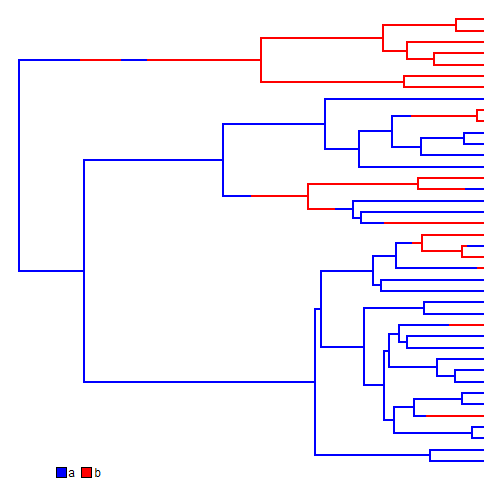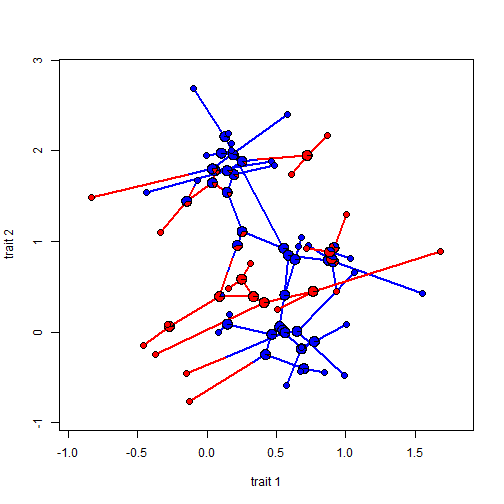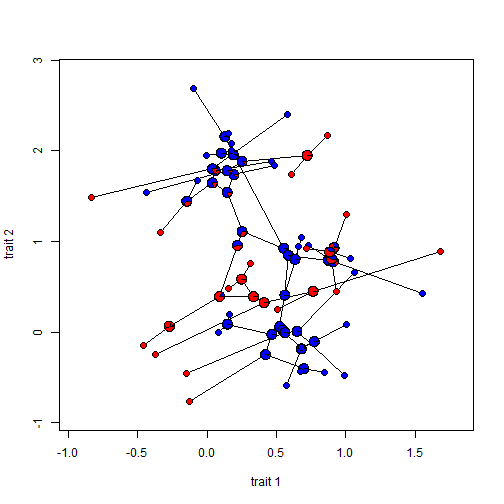## Thursday, August 20, 2015

### Adding node labels to a phylomorphospace plot

I just posted an update to the phytools function `phylomorphospace` which now sets the environmental variable `"last_plot.phylo"` so that `phylomorphospace` can now be more easily combined with ape functions such as `nodelabels` and `tiplabels`.

For instance, imagine we have a discrete character that we want to overlay on a tree projected into morphospace using the function `phylomorphospace`. At present, we can use stochastic mapping style trees of class `"simmap"` to project a single, reconstructed discrete character history. However, with `nodelabels` we can also/instead show posterior probabilities at nodes. So, for example:

``````library(devtools)
install_github("liamrevell/phytools",quiet=TRUE)
library(phytools)
## simulate bivariate data with different covariance structure
## depending on a discrete character
a<-matrix(c(1,0,0,1),2,2)
b<-matrix(c(2,1.8,1.8,2),2,2)
dimnames(a)<-dimnames(b)<-
list(c("trait 1","trait 2"),c("trait 1","trait 2"))
V<-list(a=a,b=b)
Q<-matrix(c(-1,1,1,-1),2,2)
rownames(Q)<-colnames(Q)<-names(V)
map.tree<-sim.history(tree<-pbtree(n=40,scale=1),Q,anc="a")
``````
``````## Done simulation(s).
``````
``````cols<-setNames(c("blue","red"),names(V))
plot(map.tree,colors=cols,ftype="off",ylim=c(-1,Ntip(tree)))
````````````X<-sim.corrs(map.tree,vcv=V)
colnames(X)<-colnames(V[])
phylomorphospace(map.tree,X,ftype="off",colors=cols,node.size=rep(0,2))
x<-map.tree\$states
x
``````
``````## t22 t23 t37 t38 t15 t31 t32 t27 t28 t26 t13 t14  t9 t20 t21  t6  t7  t8
## "a" "a" "a" "a" "b" "a" "a" "a" "a" "a" "a" "a" "b" "a" "a" "a" "a" "b"
## t33 t34 t19  t4  t5  t2 t16 t17  t3 t18 t35 t36 t39 t40  t1 t10 t11 t24
## "b" "a" "b" "b" "a" "a" "a" "b" "a" "a" "a" "a" "b" "b" "a" "b" "b" "b"
## t25 t12 t29 t30
## "b" "b" "b" "b"
``````
``````obj<-ace(x,tree,type="discrete",model="ER")
nodelabels(pie=obj\$lik.anc,piecol=cols,cex=0.6)
tiplabels(pie=to.matrix(x,names(V)),piecol=cols,cex=0.4)
````````````## or, alternatively:
phylomorphospace(tree,X,ftype="off",node.size=rep(0,2))
nodelabels(pie=obj\$lik.anc,piecol=cols,cex=0.6)
tiplabels(pie=to.matrix(x,names(V)),piecol=cols,cex=0.4)
``````That's it.